Vectors and conics

This free course is available to start right now. Review the full course description and key learning outcomes and create an account and enrol if you want a free statement of participation.

Free course

1.10 Further exercises

Example 12

Determine the equation of the line through each of the following pairs of points. Show that both equations can be written in the form ax + by = c, for some real numbers a, b and c, where a and b are not both zero.

• (a) (−2, −4) and (1, 6).

• (b) (0, 0) and (7, 3).

• (a) Since (−2, −4) and (1, 6) lie on the line, its gradient is

•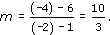• It follows that the equation of the line is

•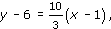• which can be simplified to

•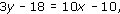• that is,

• 10x − 3y = −8.

• This equation is of the desired form, with a = 10, b = −3 and c = −8. (Any multiple of these numbers is also a valid answer.)

• (b) Since the line passes through the origin and the point (7, 3), it has an equation of the form y = mx, for some m. The coordinates of (7, 3) must satisfy the equation y = mx. Thus 3 = 7m, so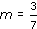.

• Hence the equation of the line is

•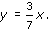• This can be written as

• 3x − 7y − 0,

• which is of the desired form, with a = 3, b = −7 and c = 0.

Example 13

Determine the values of k for which the lines 3x + 4y + 7 = 0 and 2x + ky = 3 are

• (a) parallel,
• (b) perpendicular.

The gradients of the lines 3 x + 4y + 7 = 0 and 2 x + ky = 3 are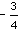and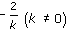, respectively.

Thus the lines are

• (a) parallel if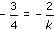, that is,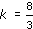;

• (b) perpendicular if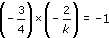, that is,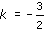.

Example 14

Sketch the lines with the following equations, on a single diagram: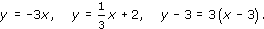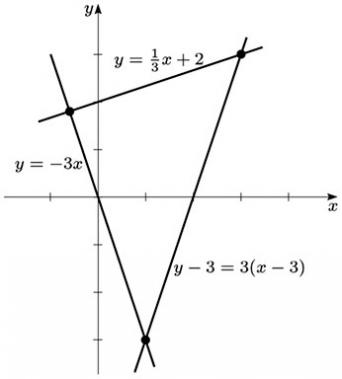Example 15

Determine the coordinates of the points of intersection of the lines in Exercise 14.

Let A be the point of intersection of the lines y = −3x and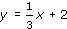. We equate the two expressions for y to obtain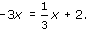Multiplying through by 3 gives

−9x = x + 6,

so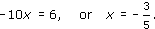Since A lies on the line y = −3x, it follows that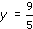So the point A has coordinates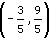Next, let B be the point of intersection of the linesand y − 3 = 3(x − 3). We rewrite the second equation, and equate the two expressions for y to obtain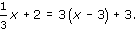Multiplying through by 3 and collecting terms gives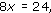so

x = 3.

Since B lies on the lineit follows that y = 3. So the point B has coordinates (3, 3).

Finally, let C be the point of intersection of the lines y = −3x and y − 3 = 3(x − 3). We rewrite the second equation, and equate the two expressions for y to obtain

−3x = 3(x − 3) + 3.

Collecting terms gives

6x = 6,

so

x = 1.

Since C lies on the line y = −3x, it follows that y = −3. So the point C has coordinates (1, −3).

Example 16

Find the distances between the vertices of the triangle formed by the points of intersection found in Exercise 15.

We use the Distance Formula given Section 1.5.

Since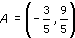, B = (3, 3) and C = (1, −3),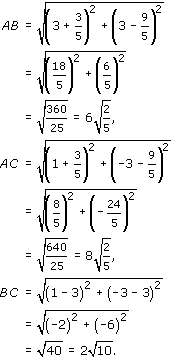Remark: In the triangle ABC,

AB2 + AC2 = BC2,

so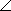BAC is a right angle.

Example 17

Determine whether each of the pairs of planes given by the following equations intersect, are parallel, or coincide.

• (a) x = 1 and y = 2.

• (b) z = 1 and z = 3.

• (a) The planes with equations x = 1 and y = 2 are parallel to the (y, z)-plane and the (x, z)-plane, respectively. They intersect in a line, as shown.

•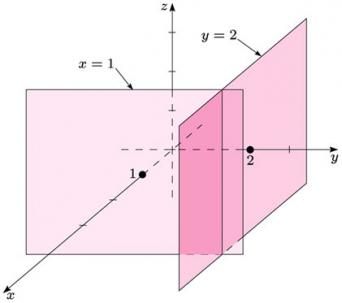•

• (b) The planes with equations z = 1 and z = 3 are both parallel to the (x, y)-plane. They are parallel to each other, as shown.

•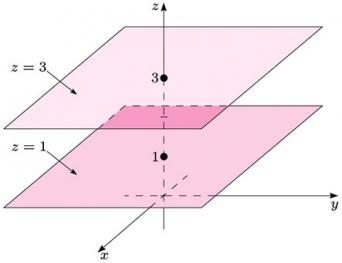Example 18

Determine the distance between the points (1, −2, 3) and (−2, 3, −1) in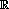3.

We use the Distance Formula given in Section 1.9. The required distance is thus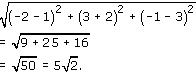M208_1